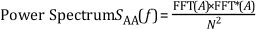# Power Spectrum

## LabVIEW 2018 Help

Edition Date: March 2018
Part Number: 371361R-01
View Product InfoLabVIEW 2016 HelpLabVIEW 2017 HelpLabVIEW 2018 HelpLabVIEW 2019 HelpLabVIEW 2020 Help

The discrete Fourier transform (DFT) or fast Fourier transform (FFT) of a real signal is a complex number, having a real and an imaginary part. You can obtain the power in each frequency component represented by the DFT or FFT by squaring the magnitude of that frequency component. Thus, the power in the kth frequency component—that is, the kth element of the DFT or FFT—is given by the following equation.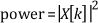where |X[k]| is the magnitude of the frequency component.

The power spectrum returns an array that contains the two-sided power spectrum of a time-domain signal and that shows the power in each of the frequency components. You can use the following equation to compute the two-sided power spectrum from the FFT.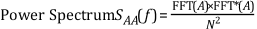where FFT*(A) denotes the complex conjugate of FFT(A). The complex conjugate of FFT(A) results from negating the imaginary part of FFT(A).

The values of the elements in the power spectrum array are proportional to the magnitude squared of each frequency component making up the time-domain signal. Because the DFT or FFT of a real signal is symmetric, the power at a positive frequency of k Δf is the same as the power at the corresponding negative frequency of -k Δf, excluding DC and Nyquist components. The total power in the DC component is |X|2. The total power in the Nyquist component is |X[N/2]|2.

A plot of the two-sided power spectrum shows negative and positive frequency components at a height given by the following relationship: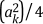where ak is the peak amplitude of the sinusoidal component at frequency k. The DC component has a height of A02 where A0 is the amplitude of the DC component in the signal.

The following figure shows the power spectrum result from a time-domain signal that consists of a 3 Vrms sine wave at 128 Hz, a 3 Vrms sine wave at 256 Hz, and a DC component of 2 VDC. A 3 Vrms sine wave has a peak voltage of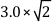or about 4.2426 V.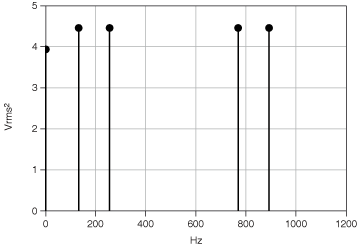The power spectrum is computed from the basic FFT function, as defined by the equation